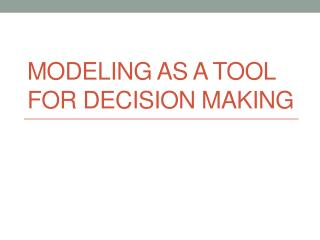DownloadDownload PresentationModeling as a tool for Decision Making

# Modeling as a tool for Decision Making

Télécharger la présentation## Modeling as a tool for Decision Making

- - - - - - - - - - - - - - - - - - - - - - - - - - - E N D - - - - - - - - - - - - - - - - - - - - - - - - - - -
##### Presentation Transcript

1. Modeling as a tool for Decision Making

2. Issues with reality • Cost • Time • Danger • Legality / Ethical / Moral • Replication • Complexity • Risk • Variability • Inability to experiment – “what if analysis”

3. Model Model is an (internal and implicit) external and explicit representation of reality as seen by the people who wish to use it and to understand, to change, to manage, and to control that reality. -Michael Pidd

4. Models • are less expensive and disruptive than experimenting with real world systems • allow “What if” questions to be asked • are built for management problems and encourage management input • enforce consistency in approach • require specific constraints and goals

5. Models Can Help … • gain deeper insight into the nature of relationships between entities or variables • find better ways to assess values in such relationships; and • see a way of reducing, or at least understanding, uncertainty that surrounds plans and actions

6. Models Can • accurately represent reality • help a decision maker understand the problem • save time and money in problem solving and decision making • help communicate problems and solutions to others • provide the only way to solve large or complex problems in a timely fashion

7. Reality vs. Models

8. Soft vs. Hard Models • Vague, ambiguous, incomplete data, confusion, lack of clarity, conflicting objectives • Precise, data availability, quantitative nature

9. Types of Models • Physical Models • Computer Models • Simulation Models • Mathematical Models • Network Models

10. Types of Mathematical Models • Deterministic models - we know all values used in the model with certainty • Probabilistic models - we know the probability that parameters in the model will take on a specific value

11. An example of a simple model Profit = Revenue - Expenses Example: Profit Profit = (Price per Unit)*(Number of Units Sold) - Fixed Cost - (Variable Costs per Unit) * (Number of Units Sold) Profit = \$10X - \$1,000 - \$5X For 1000 units Profit = \$10*(1000) - \$1,000 - \$5*(1000) = \$10,000 - \$1,000 - \$5,000 = \$4,000

12. The Downside of Models • may be expensive and time-consuming to develop and test • are often misused and misunderstood (and feared) because of their mathematical complexity • tend to downplay the role and value of nonquantifiable information • often have assumptions that oversimplify the variables of the real world

13. Possible problems in using Models • Defining the Problem • Conflicting viewpoints • Departmental impacts • Assumptions • Problems/Solutions become quickly outdated • Developing a Model • Fitting the Model • Understanding the Model • Acquiring Input Data • Accounting Data • Validity of Data

14. Possible problems in using Models… • Developing a Solution • Complex Mathematics • Only One Answer is Limiting • Test the Solution • Identifying appropriate test procedures • Analyze the Results • Holding all other conditions constant • Identifying cause and effect • Implement the Solution • Selling the solution to others

15. Approaches to Decision Making • Rational / Logical • Other • Seat of pants approach • Superstitions / Traditions / Customs • Faith / Beliefs / Trust • Do nothing

16. Summary • Modeling as a tool for decision making • Types of Models • Approaches to decision making